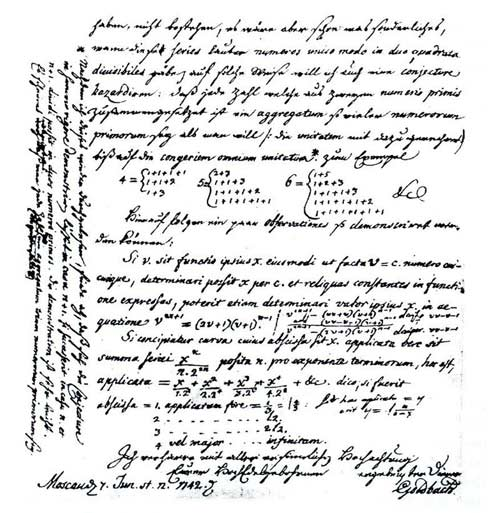A# Goldbach conjectureGoldbach's latter to Euler dated June 6, 1742.

The Goldbach conjecture is one of the oldest and easiest-to-understand hypotheses in mathematics that remains unproven. In its original form, now known as the weak Goldbach conjecture, it was put forward by the Prussian amateur mathematician and historian Christian Goldbach (1690–1764) in a letter dated 7 June 1742, to Leonhard Euler. In this guise it says that every whole number greater than 5 is the sum of three prime numbers. Euler restated this, in an equivalent form, as what is now called the strong Goldbach conjecture or, simply, the Goldbach conjecture: every even number greater than 2 is the sum of two primes. Thus, 4 = 2 + 2, 6 = 3 + 3, 8 = 3 + 5, 10 = 3 + 7, ..., 100 = 53 + 47, ...

In fact René Descartes knew about the two-prime version of Goldbach's conjecture before either Goldbach or Euler did. So, is it misnamed? Paul Erdös said, "It is better that the conjecture be named after Goldbach because, mathematically speaking, Descartes was infinitely rich and Goldbach was very poor." In any event, there is a much more important question, namely, is the conjecture true? The general assumption is that it is, but no one knows for sure. The most significant step toward a proof came in 1966 when the Chinese mathematician Chen Jing-Run showed that every sufficiently large even integer is the sum of a prime and a number that has at most two prime factors. Using powerful computers, the Goldbach conjecture has been checked out to about 400 trillion. But there is no great optimism among mathematicians that a final breakthrough is on the horizon. Even a reward of \$1 million dollars for a proof offered by the publishing house Faber & Faber in 2000, to help publicize the novel Uncle Petros and Goldbach's Conjecture by the Greek mathematician and author Apostolos Doxiadis, went unclaimed.1

### Reference

1. Doxiadis, Apostolos K. Uncle Petros and Goldbach's Conjecture. New York: Bloomsbury, 2000.